4WX Internet Search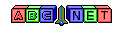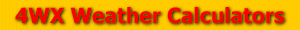# Pressure Conversion

From the user, a value for atmospheric pressure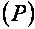is given to be converted to different pressure units.

To convert between inches of mercury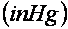and millimeters of mercury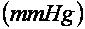or torr, use the formulas below:To convert between inches of mercuryand millibars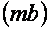or hectopascals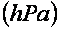, use the formulas below:To convert between inches of mercuryand kilopascals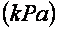, use the formulas below:To convert between inches of mercuryand pounds per square inch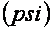, use the formulas below:To convert between torr or millimeters of mercury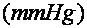and millibarsor hectopascals, use the formulas below:To convert between torr or millimeters of mercuryand kilopascals, use the formulas below:To convert between torr or millimeters of mercuryand pounds per square inch, use the formulas below:To convert between millibarsor hectopascalsand kilopascals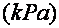, use the formulas below: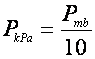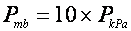To convert between millibarsor hectopascalsand pounds per square inch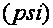, use the formulas below:To convert between kilopascalsand pounds per square inch, use the formulas below:Back to 4WX Weather Calculators index page

4WX Calculators Credit and disclaimer